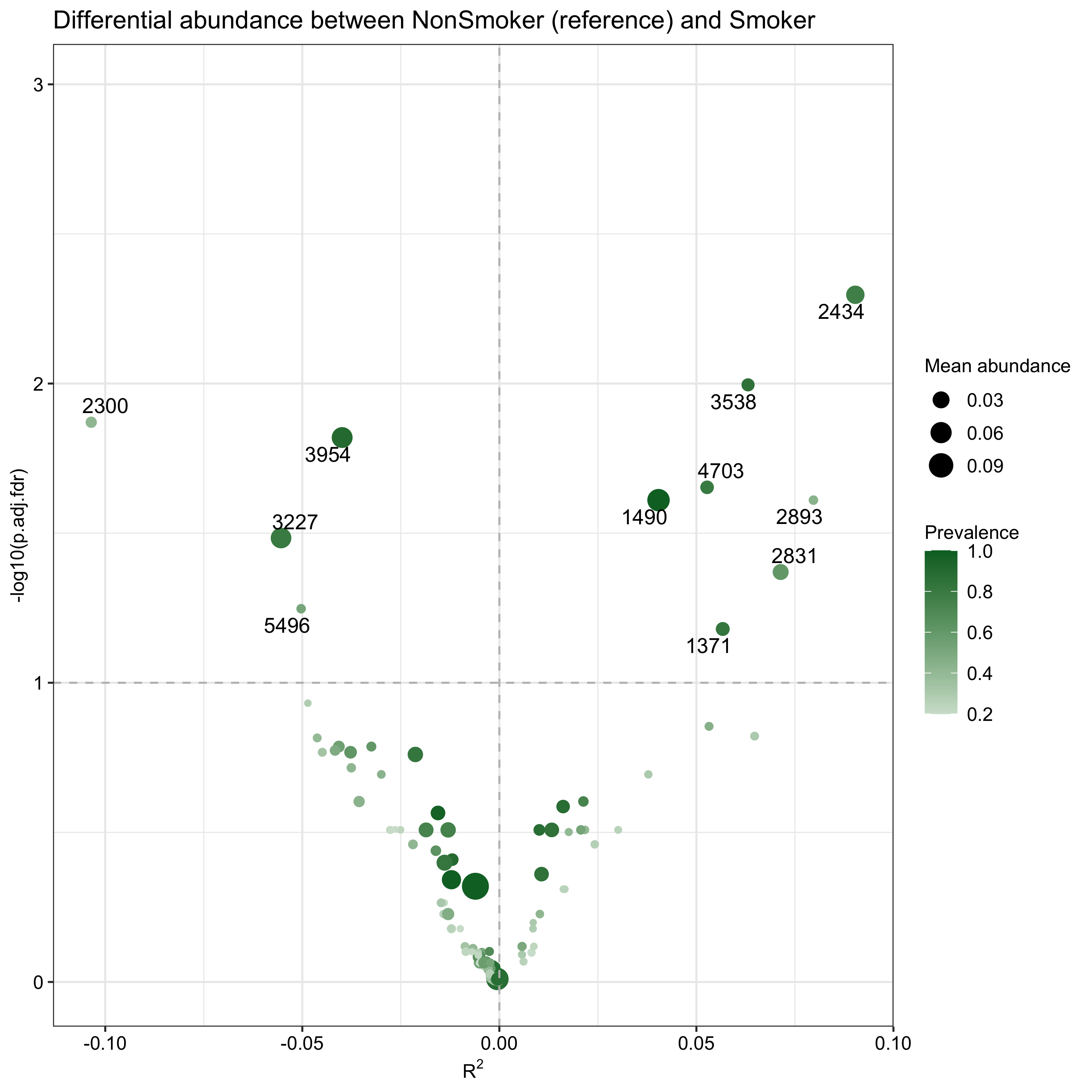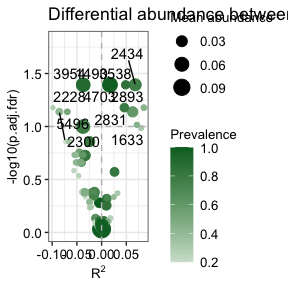# 1. Introduction

`ZicoSeq` is a linear model and permutation-based method for dfferential abundance analysis of zero-inflated compositional sequencing data such as microbiome sequencing data. It has the following components:

• Winsorization to decrease the influence of outliers;
• Posterior sampling based on a beta mixture prior to address sampling variability and zero inflation;
• Reference-based multiple-stage normalization to address compositional effects;
• An omnibus test to address diverse feature-covariate relationships;
• Permutation-based false discovery rate control / family-wise error rate control for multiple testing correction, which takes into account the correlation structure in the feature data.

Currently `ZicoSeq` supports:

• count data or proportion data. For both count and proportion data, a reference-based ratio approach is used to account for compositional effects. When a count matrix is provided, it provides an option to draw posterior samples of the underlying proportions to account for the sampling variability during the sequencing process. The test results are aggregated over these posterior samples.

• other data types. As a general methodology, `ZicoSeq` can be used to differential analysis of other high-dimensional datasets such as transcriptomics, epigenomics, metabolomics, and proteomics data.

# 2. Installation

Install the package.

``# install.packages("GUniFrac")``

``library(GUniFrac)``

# 3. Running `ZicoSeq`

This example data set contains the OTU count data from the throat microbiome of the left body side, which is available in `GUniFrac` package. It contains 60 subjects consisting of 32 nonsmokers and 28 smokers (Charlson, Emily S., et al., 2010).. In this example, we will identify smoking-associated OTUs while adjusting the sex since sex is a potential confounder (we see more males in smokers).

``````data(throat.otu.tab)
data(throat.meta)
comm <- t(throat.otu.tab)
meta.dat <- throat.meta
meta.dat``````

## 3.2 Running `ZicoSeq` function

``````ZicoSeq.obj <- ZicoSeq(meta.dat = meta.dat, feature.dat = comm,
grp.name = 'SmokingStatus', adj.name = 'Sex', feature.dat.type = "count",
# Filter to remove rare taxa
prev.filter = 0.2, mean.abund.filter = 0,
max.abund.filter = 0.002, min.prop = 0,
# Winsorization to replace outliers
is.winsor = TRUE, outlier.pct = 0.03, winsor.end = 'top',
# Posterior sampling
is.post.sample = TRUE, post.sample.no = 25,
# Use the square-root transformation
link.func = list(function (x) x^0.5), stats.combine.func = max,
# Permutation-based multiple testing correction
perm.no = 99,  strata = NULL,
# Reference-based multiple stage normalization
ref.pct = 0.5, stage.no = 6, excl.pct = 0.2,
# Family-wise error rate control
is.fwer = TRUE, verbose = TRUE, return.feature.dat = TRUE)``````

## 3.3 Some notes on using `ZicoSeq`

### 3.3.1 Winsorization

Microbiome data may contain outliers with extremely large counts. These outliers could reduce the statistical power, or more seriously increase the type I error rate. In `ZicoSeq`, we use winsorization to replace those extremely large counts with a certain quantile (e.g. 97%). We recommend always setting `is.winsor = TRUE` for real data analysis. The specific quantile used depends on the expected proportion of outliers.

### 3.3.2 Posterior sampling

Posterior sampling can be enabled by setting `is.post.sample = TRUE`. It can only be used when the data type is “count”. For microbiome data, the majority of the taxa are rare, resulting in a large number of zeros in the data given a limited sequencing depth. As the probability of zero strongly depends on the sequencing depth, zeros from different samples are not comparable. In `ZicoSeq`, we provide a method to infer/impute the underlying true proportions using an empirical Bayes approach by pooling information across samples. The imputed proportions depend on both the sequencing depth and the estimated (prior) distribution of the proportions across samples. To better model the prior distribution, we used beta mixtures instead a single-component beta distribution. Posterior sampling effectively reduces the type I error when the sequencing depth is associated with the covariate of interest (e.g. cases and controls differ in sequencing depth) and is more powerful than rarefaction. When the sequencing depth is not a confounding factor, `is.post.sample` slightly improves the power and could be disabled for analyzing large datasets.

### 3.3.3 Omnibus testing

The relationship between the taxa abundance and the covariate of interest may vary across taxa, a single transformation function may not be powerful enough to capture diverse relationships. `ZicoSeq` allows specifying multiple possible transformation functions (`link.func`) and performs omnibus testing. Although the log transformation is commonly used for microbiome due to its interpretability, we found that power transformations may be more powerful. The default is the square-root transformation.

### 3.3.4 Reference selection

`ZicoSeq` uses a reference-based approach to address compositional effects. The default uses 30% of the least variable/significant taxa as the reference. It starts with selecting 50% of the least variable taxa as the reference and differential abundance testing is then run multiple times excluding 20% taxa with the smallest p-values in each iteration. This procedure is to make sure the remaining 30% are more likely to be non-differential.

### 3.3.5. Error control

`ZicoSeq` uses a permutation-based approach to control false discovery rate (FDR). The permutation-based FDR control preserves the correlation structure in the abundance data and is more robust and powerful than the traditional BH-based FDR control based on raw p values. `ZicoSeq` also allows performing permutation-based family-wise error rate control. Users can determine the appropriate error control method to suit their needs.

## 3.4 `ZicoSeq` output

The output of `ZicoSeq` consists of mainly:

### 3.4.1 p-values

• `p.raw`: raw p-values based on permutations, it will not be accurate if `perm.no` is small.
• `p.adj.fdr`: permutation-based FDR-adjusted p-values.
• `p.adj.fwer`: permutation-based FWER-adjusted (West-Young), only if `is.fwer = T` in `ZicoSeq`. `perm.no` is recommened to set at least 999 for accurate FWER-adjusted p-values.

### 3.4.2 R^2 (percentage of variance explained)

A matrix of R^2 values (number of features by number of transformation functions) will be provided. R^2 is an effect size measure and can be used to assess the association strength between the taxa abundance and the covariate of interest while adjusting for other covariates. When the omnibus testing is in action, i.e., multiple transformations are used, there will be multiple R^2 s, each corresponding to a specific transformation.

## 3.5 `ZicoSeq` output visualization

`ZicoSeq.plot` function produces a volcano plot with the y-axis being the log10 (adjusted) p-value and the x-axis being the signed R^2 with the sign indicating the association direction determined based on the sign of the regression coefficient (for mutli-categorical variables, sign is not applicable). When multiple transformation functions are used, the largest R^2 is used. The names of differential taxa passing a specific significance cutoff will be printed on the figure. When data types are counts and proportions, the mean abundance and prevalence will be visualized; when the data type is `other`, mean and standard deviation of the features will be visualized. Users need to set `return.feature.dat = T` when using the plot function.

``````ZicoSeq.plot(ZicoSeq.obj, pvalue.type = 'p.adj.fdr', cutoff = 0.1, text.size = 10,
out.dir = NULL, width = 10, height = 6)``````## 3.6 More examples

### 3.6.1 Proportion data

For some bioinformatics pipelines, the output could be proportion data. `ZicoSeq` can be applied to proportion data by specifying `feature.dat.type = "proportion"` . Posterior sampling will not be applied when analyzing the proportions and other parameter settings are similar to the count case.

``````comm.p <- t(t(comm) / colSums(comm))
ZicoSeq.obj.p <- ZicoSeq(meta.dat = meta.dat, feature.dat = comm.p,
grp.name = 'SmokingStatus', adj.name = 'Sex', feature.dat.type = "proportion",
# Filter to remove rare taxa
prev.filter = 0.2, mean.abund.filter = 0,  max.abund.filter = 0.002, min.prop = 0,
# Winsorization to replace outliers
is.winsor = TRUE, outlier.pct = 0.03, winsor.end = 'top',
# Posterior sampling will be automatically disabled
is.post.sample = FALSE, post.sample.no = 25,
# Use the square-root transformation
link.func = list(function (x) x^0.5, function (x) x^0.25), stats.combine.func = max,
# Permutation-based multiple testing correction
perm.no = 99,  strata = NULL,
# Reference-based multiple stage normalization
ref.pct = 0.5, stage.no = 6, excl.pct = 0.2,
# Family-wise error rate control
is.fwer = TRUE, verbose = TRUE, return.feature.dat = T)
suppressWarnings(ZicoSeq.plot(ZicoSeq.obj = ZicoSeq.obj.p, pvalue.type = 'p.adj.fdr',
cutoff = 0.1, text.size = 10, out.dir = NULL, width = 10, height = 6))                    ``````### 3.6.2 General data types

`ZicoSeq` as a general linear model-based permutation test can be applied to association analyses of other high-dimensional datasets such as transcriptome-, methylome-, metabolome- and proteome-wide association testing, where the omics features are treated as the outcomes. In this case, posterior sampling will be automatically disabled. The user should be responsible for properly normalizing, transforming, and filtering the data before applying `ZicoSeq`. The user should also decide whether to winsorize the top, bottom or both ends of the distribution. The following code is just for demonstration purposes and does not mean to be applied for differential abundance analysis.

``````comm.o <- comm[rowMeans(comm != 0) >= 0.2, ] + 1
comm.o <- log(t(t(comm.o) / colSums(comm.o)))
ZicoSeq.obj.o <- ZicoSeq(meta.dat = meta.dat, feature.dat = comm.o,
grp.name = 'SmokingStatus', adj.name = 'Sex', feature.dat.type = "other",
# Filter will not be applied
prev.filter = 0, mean.abund.filter = 0,  max.abund.filter = 0, min.prop = 0,
# Winsorization the top end
is.winsor = TRUE, outlier.pct = 0.03, winsor.end = 'top',
# Posterior sampling will be automatically disabled
is.post.sample = FALSE, post.sample.no = 25,
# Identity function is used
link.func = list(function (x) x), stats.combine.func = max,
# Permutation-based multiple testing correction
perm.no = 99,  strata = NULL,
# Reference-based multiple-stage normalization will not be performed
ref.pct = 0.5, stage.no = 6, excl.pct = 0.2,
# Family-wise error rate control
is.fwer = TRUE, verbose = TRUE, return.feature.dat = T)
ZicoSeq.plot(ZicoSeq.obj = ZicoSeq.obj.o,  pvalue.type = 'p.adj.fdr',
cutoff = 0.1, text.size = 10, out.dir = NULL, width = 10, height = 6)    ``````